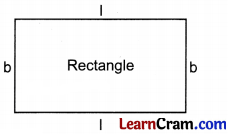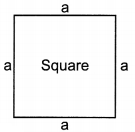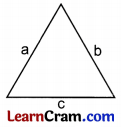# DAV Class 4 Maths Chapter 11 Worksheet 3 Solutions

The DAV Maths Book Class 4 Solutions and DAV Class 4 Maths Chapter 11 Worksheet 3 Solutions of Perimeter offer comprehensive answers to textbook questions.

## DAV Class 4 Maths Ch 11 WS 3 Solutions

Question 1.
Solve the following word problems.
(а) Find the perimeter of a rectangle whose length is 12 cm and breadth is 8 cm.
Perimeter of a rectangle = 2 l + 2 b
= 2 × 12 cm + 2 × 8 cm
= 24 cm + 16 cm
= 40 cm
Perimeter = 40 cm

(b) Calculate the perimeter of the square whose sides are given below.
(i) 6 cm
(ii) 11 meters
(iii) 26 cm
(i) Perimeter of square = 4 × side = 4 × 6 cm
= 24 cm.
(ii) Perimeter of square = 4 × side = 4 × 11 m
= 44 m.
(iii) Perimeter of square = 4 × side = 4 × 26 cm
= 104 cm.

(c) The length of three sides of a triangle are 6 cm, 8 cm and 10 cm. Find its perimeter.
Perimeter of triangle = sum of three sides
= 6 cm + 8 cm + 10 cm
= 24 cm.

(d) A boy runs around a rectangular field, the length of which is 35 metres and the breadth is 20 metres. How much distance will be run?
To find the distance, we have to find perimeter of rectangular field.
Length of rectangular field = 35 m
Breadth of rectangular field = 20 m
Perimeter of field = 2 × 35m + 2 × 20m
= 70 m + 40 m = 110 m
Boy will run 110 m.

(e) Sheela wants to fix ribbon along the border of a painting. If the length and breadth of the painting are 36 cm and 15 cm respectively how much ribbon is required by Sheela?
Since the length and breadth is different so shape of frame is rectangle so we have to find perimeter of frame.
Length of frame = 36 cm
Breadth of frame = 15 cm
Perimeter of frame = 2 × 36cm + 2 × 15cm
= 72 cm + 30 cm
= 102 cm
102 cm ribbon is required.(f) Raju has to frame a square photo having one side of length 12 cm. How much material will Raju require for this job?
We have to find the perimeter of square photo.
Perimeter of square frame = 4 × side
= 4 × 12 cm
= 48 cm
48 cm material is required.

(g) A boy runs two times around a field, the length of which is 62 m and breadth is 40 m. Find the distance covered by him.
We have to find perimeter of field.
Length of field = 62 m
Breadth of field = 40 m
Perimeter of the field = 2(62 + 40)
= 2(102)
= 204 m
He runs 2 times so distance covered = 2 × 204 m
= 408 m.

(h) Tina ran around a rectangular garden of length 12 m and breadth 8 m. Meena ran around a square field of side 11m. Who covered more distance and by how much?
In both cases we have to find distance means perimeter.
Distance covered by Tina around a rectangular garden = 2 × 12 m + 2 × 8 m
= 24 m + 16 m
= 40 m
Distance covered by Meena, around a square field = 4 × side
= 4 × 11 m
= 44 m
Hence, Meena covered more distance.
Extra distance = 44 – 40
= 4 m

### DAV Class 4 Maths Chapter 11 Worksheet 3 Notes

Perimeter of Rectangle, Square And Triangle
(i) Rectangle:
Opposite sides of the rectangle are equal so the perimeter will be
= l + b + l + b
= 2l + 2 b
= 2 (l + b) unitLet l = 3 cm, b = 2 cm,
Perimeter 2(3 + 2)
= 10 cm

(ii) Square:
Let the side of square be a since all the sides are equal.
The perimeter = a + a + a + a.
= 4a unit
Let side = 4 cm
Perimeter = 4 × 4
= 16 cm(iii) Triangle
Perimeter of the triangle will be sum of three sides.
Let the sides be a, b, and c
Perimeter = a + b + c
Let a = 2cm, b = 3cm and c = 4cm
Perimeter = 2 + 3 + 4
= 9 cm.Question 1.
Babita’s grandfather has a rectangular garden whose length is 70 m and breadth is 40 metres. How much wire is needed to fence the field?
The length of wire needed will be equal to perimeter of field.
Length of field = 70 m
Breadth of field = 40 m
Length of wire needed = 2 × 70m + 2 × 40m
= 140 m + 80 m
= 220 m
220 m wire is needed.

Question 2.
Meena wants to fix a border of a square carpet whose each side is 9 m. Find the length of border.